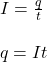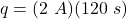## in the circuit diagram below on voltmeter V2 is 12V and the reading on ammeter A1 is 2A calculate the amount of charge that flows thr

Question

in the circuit diagram below on voltmeter V2 is 12V and the reading on ammeter A1 is 2A
calculate the amount of charge that flows through ammeter A1 in 120 second​

in progress 0
2 weeks 2021-09-03T17:27:06+00:00 1 Answers 0 views 0

q = 240 C

Explanation:

The current passing through a circuit is defined as the amount of charge passing through that circuit per unit of time. Hence, the current can be calculated through the following formula:where,

q = total amount of charge = ?

I = current = 2 A

t = time = 120 s

Therefore,q = 240 C